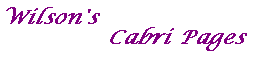#### Proof of Theorem 5

Theorem 5
Suppose that C: xTMx=0 and D: xTNx=0 are non-degenerate conics.
Then the dual of D with respect to C is the non-degenerate conic with equation xTMN-1Mx=0.

Proof
We think of D as the line-conic (the envelope of its tangents), {uTNx=0 : uTNu=0}.
The dual of uTNx=0 with respect to C is the point V=[v], where v= M-1(Nu).
Then u= N-1(Mv).
Since [u] is on D, uTNu=0, and so vTMN-1NN-1Mv=0, i.e. vTMN-1Mv=0.
Thus the dual of D with respect to C has equation xTMN-1Mx=0, as required.